## 4.9.2 Convergence of the Self-Consistant Simulations

The coupled system of transport and POISSON equation can be solved by iteration with appropriate numerical damping, which terminates if a convergence criterion is satisfied . In this work, the maximum element of the potential update, corresponding to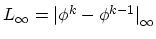, is considered as a measure of convergence.

One of the reasons causing convergence problems [256,257] is the exponential dependence of the carrier concentration on the electrostatic potential,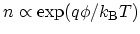. A small potential variation causes large variation in the carrier concentration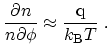(4.62)

As a result, a strong damping is required in many cases, which increases the simulation time. To avoid this problem a non-linear POISSON equation is generally employed . Solving a non-linear POISSON equation takes that exponential dependence into account. Compared to the linear POISSON equation, it leads to faster convergence in both semi-classical  and quantum mechanical [259,257] transport simulations. In this work the GUMMEL method along with a non-linear POISSON equation is employed.

However, we show that an inappropriate energy grid for the discretization of the transport equations can be another reason of convergence problems in quantum transport simulations. It is demonstrated that with adaptive energy grids the iterative solution can converge very fast and the simulation time can decrease considerably.

In Section 4.8.2 it was shown that with a shift of an equidistant grid the calculated carrier concentration can change sharply. This sensitivity resulting from the numerical error causes convergence problems in the self-consistent loop. In all non-adaptive methods some fixed energy grid is adopted. In successive iterations of the POISSON and transport equations, the electrostatic potential changes and this in turn affects the relative distance between resonance energies and the energy grid points. As a result, the evaluated carrier concentration can vary sharply in one iteration step, which affects the calculated electrostatic potential for the next iteration. For a quantitative analysis one can assume that the shift of energy grid is due to the variation of the electrostatic potential,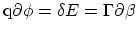.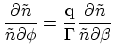(4.63)

The sensitivity of the calculated carrier concentration with respect to energy grid shifts defined by (4.63) is shown in Fig. 4.12. For a relatively coarse grid,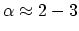, the sensitivity (4.63) can be approximated as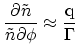(4.64)

In this case the contribution of a resonance of width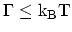will be larger than (4.62). To reduce the effect of this term, a fine grid spacing must be used (). The non-adaptive method requires many grid points to resolve fine resonances, while the adaptive method puts many energy grid points only close to resonances and few ones off the resonance. Therefore, the adaptive method keeps the total number of grid points quite low and maintains a high accuracy.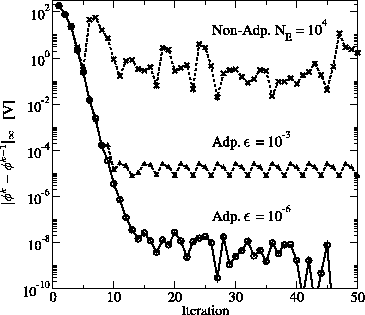The convergence of the self-consistent loop using the adaptive and non-adaptive methods is studied. With the non-adaptive method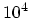energy grid points are used. For the adaptive method, relative errors of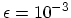and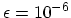are assumed. Fig. 4.16 shows the infinity norm of the potential update after each iteration. With the adaptive method the norm of the potential update decreases exponentially and finally reaches a limit which depends on the error tolerance of the integration. With the non-adaptive method the norm of the potential update oscillates and no convergence is achieved. Fig. 4.17 shows the calculated carrier concentration due to several confined states, based on four successive iterations of the non-adaptive method. From the first to the second iteration the carrier concentration changes very sharply. Therefore, at the first iteration one is close to the highly sensitive region (see Fig. 4.12). From the second to the third iteration the carrier concentration changes only little, which can be mapped to the low sensitive region. From the third to the forth iteration the variation is large, which implies that we are again close to the highly sensitive region. This sequence continues and prevents convergence. To avoid this problem a fine grid spacing can be used, which decreases the sensitivity in all regions. As it was shown in Fig. 4.10 the non-adaptive method requires a grid spacing smaller than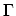for accurate result.

By reducingtoin the adaptive method the self-consistent iteration yields more accurate results, but the number of required energy grid points increases, which increases the simulation time of each iteration. Fig. 4.18 shows the infinity norm of the potential update versus CPU-time. A suitable criterion for the termination of the self-consistent loop was found as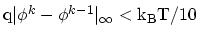. If the maximum potential update in the device is much smaller than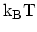, the carrier concentration will change only weakly during the next iteration. For most of the simulations performed such a criterion was satisfied for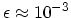.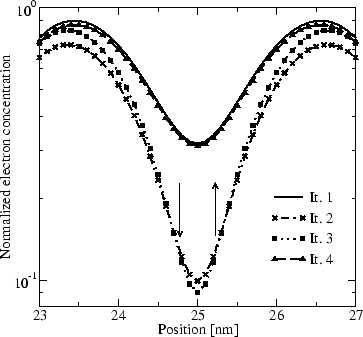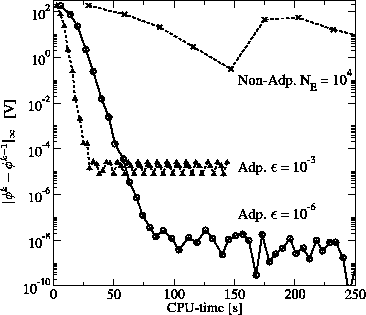M. Pourfath: Numerical Study of Quantum Transport in Carbon Nanotube-Based Transistors